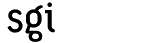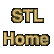# find_endCategory: algorithms Component type: function

### Prototype

find_end is an overloaded name; there are actually two find_end functions.
```template <class ForwardIterator1, class ForwardIterator2>
ForwardIterator1
find_end(ForwardIterator1 first1, ForwardIterator1 last1,
ForwardIterator2 first2, ForwardIterator2 last2);

template <class ForwardIterator1, class ForwardIterator2,
class BinaryPredicate>
ForwardIterator1
find_end(ForwardIterator1 first1, ForwardIterator1 last1,
ForwardIterator2 first2, ForwardIterator2 last2,
BinaryPredicate comp);
```

### Description

Find_end is misnamed: it is much more similar to search than to find, and a more accurate name would have been search_end.

Like search, find_end attempts to find a subsequence within the range [first1, last1) that is identical to [first2, last2). The difference is that while search finds the first such subsequence, find_end finds the last such subsequence. Find_end returns an iterator pointing to the beginning of that subsequence; if no such subsequence exists, it returns last1.

The two versions of find_end differ in how they determine whether two elements are the same: the first uses operator==, and the second uses the user-supplied function object comp.

The first version of find_end returns the last iterator i in the range [first1, last1 - (last2 - first2)) such that, for every iterator j in the range [first2, last2), *(i + (j - first2)) == *j. The second version of find_end returns the last iterator i in [first1, last1 - (last2 - first2)) such that, for every iterator j in [first2, last2), binary_pred(*(i + (j - first2)), *j) is true. These conditions simply mean that every element in the subrange beginning with i must be the same as the corresponding element in [first2, last2).

### Definition

Defined in the standard header algorithm, and in the nonstandard backward-compatibility header algo.h.

### Requirements on types

For the first version:
• ForwardIterator1 is a model of Forward Iterator.
• ForwardIterator2 is a model of Forward Iterator.
• ForwardIterator1's value type is a model of EqualityComparable.
• ForwardIterator2's value type is a model of EqualityComparable.
• Objects of ForwardIterator1's value type can be compared for equality with Objects of ForwardIterator2's value type.
For the second version:
• ForwardIterator1 is a model of Forward Iterator.
• ForwardIterator2 is a model of Forward Iterator.
• BinaryPredicate is a model of Binary Predicate.
• ForwardIterator1's value type is convertible to BinaryPredicate's first argument type.
• ForwardIterator2's value type is convertible to BinaryPredicate's second argument type.

### Preconditions

• [first1, last1) is a valid range.
• [first2, last2) is a valid range.

### Complexity

The number of comparisons is proportional to (last1 - first1) * (last2 - first2). If both ForwardIterator1 and ForwardIterator2 are models of Bidirectional Iterator, then the average complexity is linear and the worst case is at most (last1 - first1) * (last2 - first2) comparisons.

### Example

```int main()
{
char* s = "executable.exe";
char* suffix = "exe";

const int N = strlen(s);
const int N_suf = strlen(suffix);

char* location = find_end(s, s + N,
suffix, suffix + N_suf);

if (location != s + N) {
cout << "Found a match for " << suffix << " within " << s << endl;
cout << s << endl;

int i;
for (i = 0; i < (location - s); ++i)
cout << ' ';
for (i = 0; i < N_suf; ++i)
cout << '^';
cout << endl;
}
else
cout << "No match for " << suffix << " within " << s << endl;
}
```

### Notes

 The reason that this range is [first1, last1 - (last2 - first2)), instead of simply [first1, last1), is that we are looking for a subsequence that is equal to the complete sequence [first2, last2). An iterator i can't be the beginning of such a subsequence unless last1 - i is greater than or equal to last2 - first2. Note the implication of this: you may call find_end with arguments such that last1 - first1 is less than last2 - first2, but such a search will always fail.